7:46 PM
Adding Velocities: A Walk on the Train Michael Fowler, UVa Physics, 12/1/07

# Adding Velocities: A Walk on the Train

Michael FowlerUVa Physics, 12/1/07

### The Formula

If I walk from the back to the front of a train at 3 m.p.h., and the train is traveling at 60 m.p.h., then common sense tells me that my speed relative to the ground is 63 m.p.h. As we have seen, this obvious truth, the simple addition of velocities, follows from the Galilean transformations.  Unfortunately, it can’t be quite right for high speeds!  We know that for a flash of light going from the back of the train to the front, the speed of the light relative to the ground is exactly the same as its speed relative to the train, not 60 m.p.h. different.  Hence it is necessary to do a careful analysis of a fairly speedy person moving from the back of the train to the front as viewed from the ground, to see how velocities reallyadd.

We consider our standard train of length L moving down the track at steady speed v, and equipped with synchronized clocks at the back and the front.  The walker sets off from the back of the train when that clock reads zero.  Assuming a steady walking speed of u meters per second (relative to the train, of course), the walker will see the front clock to read L/u seconds on arrival there.

How does this look from the ground?  Let’s assume that at the instant the walker began to walk from the clock at the back of the train, the back of the train was passing the ground observer’s clock, and both these clocks (one on the train and one on the ground) read zero.  The ground observer sees the walker reach the clock at the front of the train at the instant that clock reads L/u (this is in agreement with what is observed on the train—two simultaneous events at the same place are simultaneous to all observers), but at this same instant, the ground observer says the train’s back clock, where the walker began, reads L/u + Lv/c2.  (This follows from our previously established result that two clocks synchronized in one frame, in which they are L apart, will be out of synchronization in a frame in which they are moving atv along the line joining them by a time Lv/c2.)

Now, how much time elapses as measured by the ground observer’s clock during the walk? At the instant the walk began, the ground observer saw the clock at the back of the train (which was right next to him) to read zero.  At the instant the walk ended, the ground observer would say that clock read L/u + Lv/c2, from the paragraph above.  But the ground observer would see that clock to be running slow, by the usual time dilation factor: so he would measure the time of the walk on his own clock to be: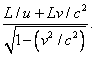How far does the walker move as viewed from the ground?  In the time tW, the train travels a distance vtW, so the walker moves this distance plus the length of the train.  Remember that the train is contracted as viewed from the ground!  It follows that the distance covered relative to the ground during the walk is:The walker’s speed relative to the ground is simply dW/tW, easily found from the above expressions: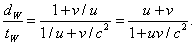This is the appropriate formula for adding velocities. Note that it gives the correct answer, u + v, in the low velocity limit, and also if u or v equals c, the sum of the velocities is c.

Exercise 1:  The direct derivation given above is really equivalent to a rederivation of the Lorentz equations. The equation describing the walker's path on the train is just x' = ut'. Substitute this in the Lorentz equations and prove it leads to a path relative to the ground given by x = wt, with w given by the velocity addition formula.

Exercise 2:  Suppose a spaceship is equipped with a series of one-shot rockets, each of which can accelerate the ship to c/2 from rest.  It uses one rocket to leave the solar system (ignore gravity here) and is then traveling at c/2 (relative to us) in deep space.  It now fires its second rocket, keeping the same direction.  Find how fast it is moving relative to us.  It now fires the third rocket, keeping the same direction.  Find its new speed.  Can you draw any general conclusions from your results?

### Walking Across the Train

Imagine now a rather wide train, of width w, and the walker begins the walk across the train, which is now equipped with clocks on both sides, when the clock where he begins reads t = 0.  For walking speed uy' (relative to the train, and across the train is the y-direction) when he reaches the clock at the other side it will read w/uy'.  How is this seen from the ground?  The width of the train w will be the same, there is no Lorentz contraction in the y-direction for motion in thex-direction.  The beginning and ending clocks will also be synchronized as seen from the ground, since they are separated in the y-direction but not the x-direction.  However, they are clocks moving at relativistic speed, so they will exhibit the familiar time dilation factor.  That is, when they read w/uy', a clock on the ground will read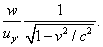Thus, as observed from the ground, walking directly across the train is slowed down by the time dilation factor, just as is every other activity on the train as seen from the ground.

However, for steady motion on the train in an arbitrary direction, velocity components (ux'uy') the cross-train velocity transforms in a more complicated way, because the train clocks at the beginning and end of the walk are now separated in the x-direction, so if they register an elapsed time of w/uy a ground observer would add a lack of synchronicity term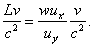Thus the time for the walk as observed from the ground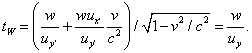From this we find the general formula for transformation of transverse velocities: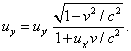For the special case of walking directly across the train, ux' = 0, we recover the earlier result, that transverse velocity is simply slowed by the time dilation effect.

### Testing the Addition of Velocities Formula

Actually, the first test of the addition of velocities formula was carried out in the 1850s!  Two French physicists, Fizeau and Foucault, measured the speed of light in water, and found it to be c/n, where n is the refractive index of water, about 1.33. (This was the result predicted by the wave theory of light.)

They then measured the speed of light (relative to the ground) in moving water, by sending light down a long pipe with water flowing through it at speed v.  They discovered that the speed relative to the ground was not just v + c/n, but had an extra term, v + c/n - v/n2.  Their (incorrect) explanation was that the light was a complicated combination of waves in the water and waves in the aether, and the moving water was only partially dragging the aether along with it, so the light didn’t get the full speed v of the water added to its original speed c/n.

The true explanation of the extra term is much simpler: velocities don’t simply add.  To add the velocity v to the velocityc/n, we must use the addition of velocities formula above, which gives the light velocity relative to the ground to be:

(v + c/n)/(1 + v/nc)

Now, v is much smaller than c or c/n, so 1/(1 + v/nc) can be written as (1 - v/nc), giving:

(v + c/n)(1 - v/nc)

Multiplying this out gives v + c/n - v/n2 -v/n×v/c, and the last term is smaller than v by a factor v/c, so is clearly negligible.

Therefore, the 1850 experiment looking for “aether drag” in fact confirms the relativistic addition of velocities formula!  Of course, there are many other confirmations.  For example, any velocity added to c still gives c.  Also, it indicates that the speed of light is a speed limit for all objects, a topic we shall examine more carefully in the next lecture.

Category: Education | Views: 461 | Added by: farrel | | Rating: 0.0/0